Courses

# NCERT Solutions (Part- 1)- Direct and Inverse Proportions Class 8 Notes | EduRev

## Class 8 Mathematics by VP Classes

Created by: Full Circle

## Class 8 : NCERT Solutions (Part- 1)- Direct and Inverse Proportions Class 8 Notes | EduRev

The document NCERT Solutions (Part- 1)- Direct and Inverse Proportions Class 8 Notes | EduRev is a part of the Class 8 Course Class 8 Mathematics by VP Classes.
All you need of Class 8 at this link: Class 8

Question 1. Observe the following tables and find if x and y are directly proportional.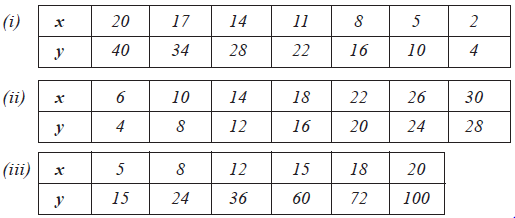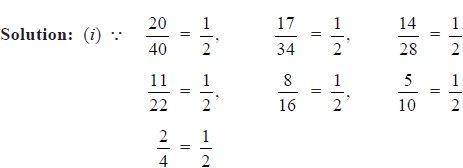i.e. Each ratio is the same.
∴ x and y are directly proportional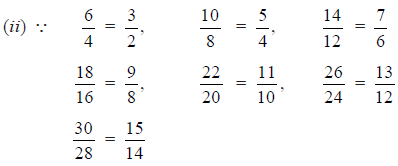i.e. All the ratios are not the same.
∴ x and y are not directly proportional.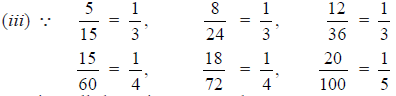i.e. All the ratios are not the same.
∴ x and y are not directly proportional.

Question 2. Principal = Rs 1000, Rate = 8% per annum. Fill in the following table and find which type of interest (simple or compound) change in direct proportion with time period.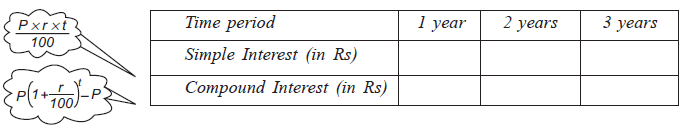Solution. Case of Simple Interest [P = Rs 1000, r = 8% p.a.]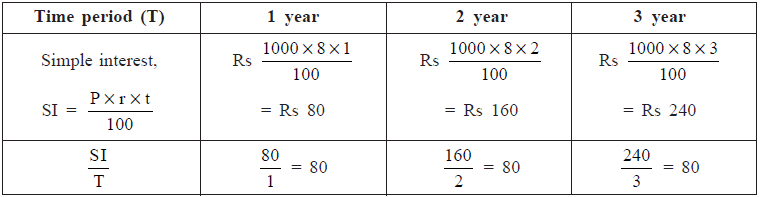∵ In each case the ratio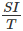is the same.
∴ The simple interest changes in direct proportion with time period.
Case of Compound Interest [P = Rs 1000, r = 8% p.a.]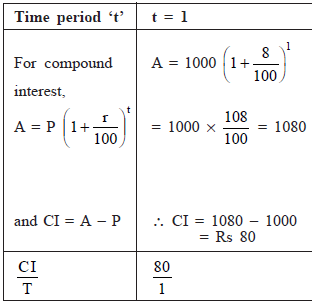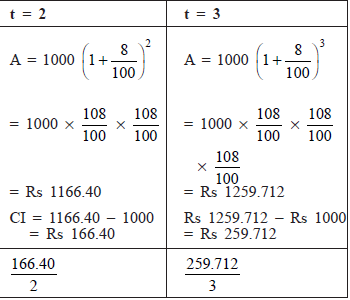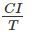is not the same in each case.
∴ The compound interest does not change in direct proportion with time period.

THINK, DISCUSS AND WRITE
Question: If we fix time period and the rate of interest, simple interest changes proportionally with principal. Would there be a similar relationship for compound interest? Why?
Solution. For simple interest,Since rate and time period are constant, then SI changes directly according to P.
Thus, the simple interest changes in direct proportion with the principal.
For compound interest,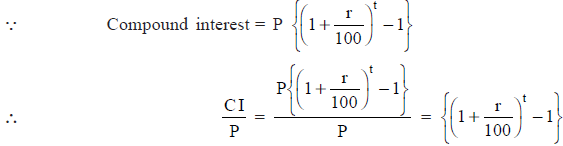Since, rate and time period are constant, then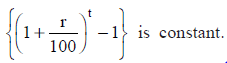i.e. CI changes with P.
Thus, the compound interest also changes directly in proportion with principal.

EXERCISE 13.1
Question 1. Following are the car parking charges near a railway station upto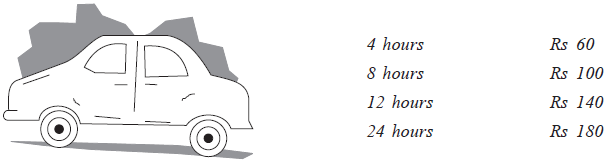Check if the parking charges are in direct proportion to the parking time.

Solution: We have: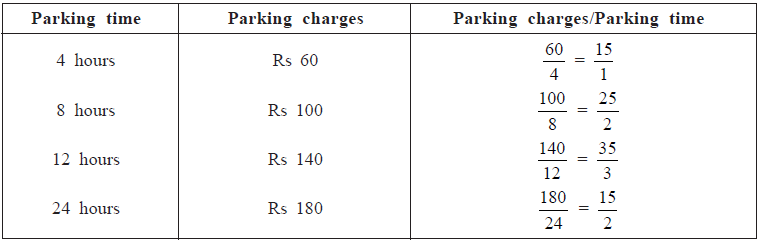Since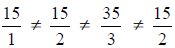∴ The parking charges are not in direct proportion with the parking time.

Question 2. A mixture of paint is prepared by mixing 1 part of red pigments with 8 parts of base. In the following table, find the parts of base that need to be added.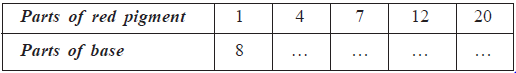Solution: Let the red pigment be represented by x1, x2, x3, … and the base represented by y1, y2, y3, ... .
As the base increases, the required number of red pigments will also increase.
∴ The quantities vary directly.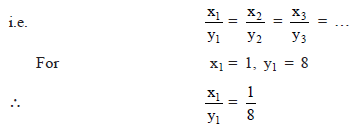Now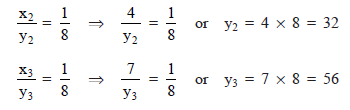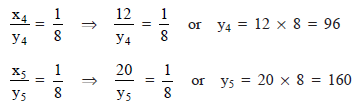Thus, the required parts of base are: 32, 56, 96 and 160.

Question 3. In question 2 above, if 1 part of a red pigment requires 75 mL of base, how much red pigment should we mix with 1800 mL of base?
Solution: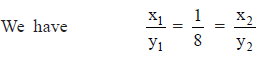Here, x1 = 1, y1 = 75 and y2 = 1800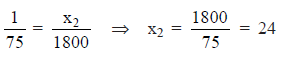Thus, the required red pigments = 24 parts.

Question 4. A machine in a soft drink factory fills 840 bottles in six hours. How many bottles will it fill in five hours?
Solution:

 Number of bottles filled Number of hours 840 6 x 5

For more number of hours, more number of bottles would be filled. Thus given quantities vary directly.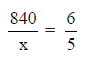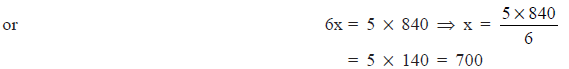Thus, the required number of bottles = 700

Question 5. A photograph of a bacteria enlarged 50,000 times attains a length of 5 cm as shown in the diagram. What is the actual length of the bacteria? If the photograph is enlarged 20,000 times only, what would be its enlarged length?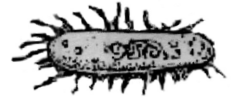Solution: Let the actual length of the bacteria = x cm

 Number of times photograph enlarged Length (in cm) 1 x 50,000 5

The length increases with an increment in the number of times the paragraph enlarged.
∴ It is a case of direct proportion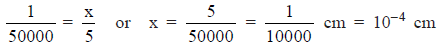Again,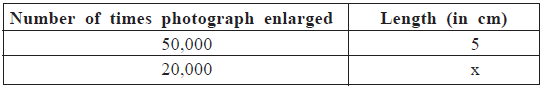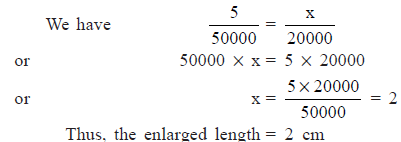Question 6. In a model of a ship, the mast is 9 cm high, while the mast of the actual ship is 12 m high. If the length of the ship is 28m, how
long is the model ship?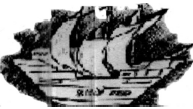Solution: Let the required length of the model of the ship = x cm
We have:

 Length of the ship Height of the mast 28 12 x 9

Since, more the length of the ship, more would be the length of its mast.
∴ It is a case of direct variation.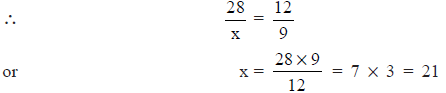Thus, the required length of the model = 21 cm

Question 7. Suppose 2 kg of sugar contains 9 * 106 crystals. How many sugar crystals are there in
(i) 5 kg of sugar? and (ii) 1.2 kg of sugar?
Solution: Let the required number of sugar crystals be x in 5 kg of sugar.
We have: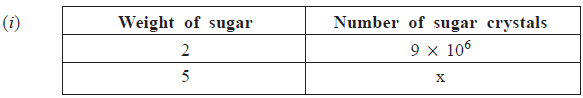Since, more the amount of sugar, more would be the number of sugar crystals.
∴  It is a case of direct variation.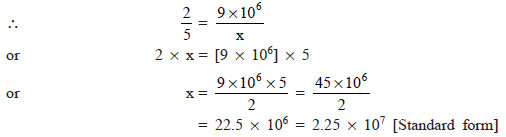Thus, the required number of sugar crystals = 2.25 * 107
(ii) Let the number sugar crystals in 1.2 kg of sugar be y.
∴ We have: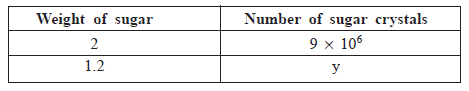∴ For a direct variation,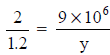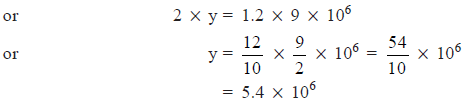Question 8. Rashmi has a road map with a scale of 1 cm representing 18 km. She drives on a road for 72 km. What would be her distance covered in the map?
Solution: Let the required distance covered in the map be x cm.
We have: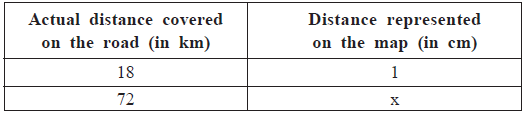Since, it is a case of direct variation,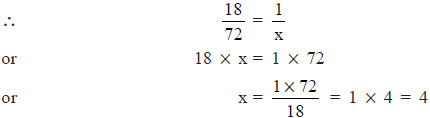Thus, the required distance on the map is 4 cm.

Question 9. A 5 m 60 cm high vertical pole casts a shadow 3 m 20 cm long. Find at the same time(i) the length of the shadow cast by another pole 10 m 50 cm high and (ii) the height of a pole which casts a shadow 5 m long.
Solution: (i) Let the required length of shadow be x cm.
We have: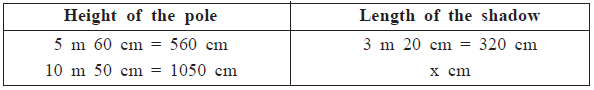As the height of the pole increases the length of its shadow also increases in the same ratio. Therefore, it is a case of direct variation.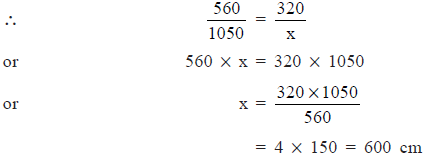Thus, the required length of the shadow = 600 cm or 6 m

(ii) Let the required height of the pole be y cm.
∴ We have: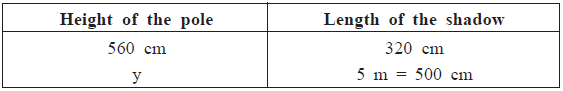Since, it is a case of direct variation,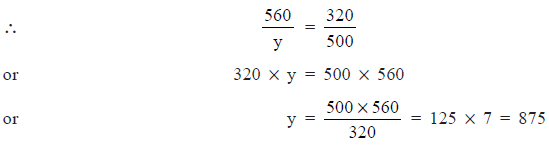Thus, the required height of the pole = 875 cm or 8 m 75 cm

Question 10. A loaded truck travels 14 km in 25 minutes. If the speed remains the same, how far can it travel in 5 hours?
Solution: Since the speed is constant,
∴ For longer distance, more time will be required.
So, it is a case of direct variation.
Let the required distance to be travelled in 5 hours (= 300 minutes) be x km.
We have:

 Distance (in km) Time (in minutes) 14x 25300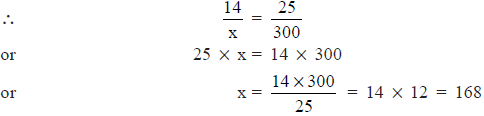∴ The required distance = 168 km

93 docs|16 tests

,

,

,

,

,

,

,

,

,

,

,

,

,

,

,

,

,

,

,

,

,

;